HOME     CALENDAR     NETWORK     FUNDING     CONTACT     RESOURCE     TOURS     SACRED GEOMETRY     ANTHROPOCOSM     EARTH GRID     VORTEX SITES     SILK ROAD     GEOMANCY     HARMONICS     ANTI-GRAVITY

[under construction]

astronomy (number in space-time) guides Harmonics, or music (number in time), within a specific geometry (number in space) calibrated/measured [eg.earth measure for specific earth grid point) by arithmetic (number in number).

calculation/divination [eg. sound healing on vortex sites (eg.temples) during specific astrological occurrences; exorcism; divination theatre; etc.].

Music is the bridge between humanity and heaven.

In acoustics and telecommunication, the harmonic of a wave is a component frequency of the signal that is an integer multiple of the fundamental frequency. For example, if the frequency is f, the harmonics have frequency 2f, 3f, 4f, etc, as well as f itself. The harmonics have the property that they are all periodic at the signal frequency. Also, due to the properties of Fourier series, the sum of the signal and its harmonics is also periodic at that frequency. (Wikipedia on 'Harmonics')

Mathematics of the World Grid: GEO-MERIDIAN THEORY

(Bruce L. Cathie; pilot and grid researcher: UFO activity in correlation with the Earth grid in New Zealand )Bruce L. Cathie adopted the cuboctahedron (sim.Fuller's VE) for the Earth Grid.

DEFINITIONS:

Harmony: a state of order, agreement, or completeness in the relations of things, or of parts of a whole to each other

Harmonic: producing, characterized by, or pertaining to, harmony

a) Music: pertaining to a tone whose rate of vibration is an exact multiple of a given primary tone

b) Mathematics: derived from, or originally suggested by, the numerical relations between the vibrations of the musical harmonies, or overtones, of the same fundamental tone; harmonic functions

c) Physics: any component of a periodic quantity, which is an integral multiple of the fundamental frequency

-Britannica World Standard Dictionary

UNIFIED FIELD THEORY: Bending Space-Time

Einstein's original theory found -untold

According to Einstein, the geometric structure of space-time determines the physical process. According to Cathie, space and time manifest from the geometric harmonies of the wave-motions of light; is there a unified field theory? (earth grid)

The fundamental harmonic of light in free space,

in geometric terms: angular velocity of 144,000 minutes of arc per grid second,

there being 97,200 grid seconds per 1 Earth revolution

(normally 24 standard periods/hours; translates as 27 grid hours, one of which is slightly shorter than a standard hour, and consists of 60 grid minutes, which consists of 60 grid seconds).

Therefore, 1 Earth revolution has:

• Standard time of 86400 seconds (24x60x60)
• Grid time of 97200 seconds (27x60x60)
• Ratio is 8:9 (86400:97200)

When physical matter is manifested in the universe, the wave-forms of light from which it is formed are slowed down fractionally in order to release the energy required for the formation process.

The harmonic of the speed of light in free space has a value of 144; for 1/2 cycle; 144/2 = 72

If this value was applied to the right triangle (3-4-5) of Pythagoras: earth grid ratio (216-288-360)

(3) 216 = 21,600 = number of arc minutes (60 min per degree) in a circle

(5) 360 = 360 = number of degrees in a circle [point activation: earth spins 15°/hour (360/24=15); 30°/bi-hour (360/12=30; zodiac)]

(4) 288 = (144x2) = 2c, where c = the speed of light harmonic [E = mc2]

The harmonic of light has a direct relationship with the geometry of the circle; as Pythagoras seems to have been aware.

According to Cathie, the primary grid lines are spaced at intervals of 7.5 minutes of arc N-S/E-W;

If there are 21,600 arc minutes in a circle, which is then divided by 7.5, then a value of 2880 results; 2880 harmonically tuned to the speed of light x2 (288)

The only way to traverse the vast distances of space without time, is to possess the means of manipulating, or altering, the structure of space; the alteration of the space-time geometric matrix (which provides the illusion of form and distance); alteration of frequencies controlling the matter-antimatter cycles, which govern the perception of space-time structure. If it was possible to move from one point in space to another (on the grid) in no time (zero-time), then both point positions would coexist in the observer's reality. Increasing the speed of geometric time decreases space proximity of place (eg.UFO: theoretically travels by means of altering surrounding spatial dimensions for repositioning in space-time; induces zero-point spin via receiving Earth Grid scalar waves transmissions).

Cathie's Harmonic Value Observations:

1703: 4-figure harmonic of 170,300,000,000 (expression in cubic minutes of arc of the mass/volume of planet Earth

1439: 4-figure harmonic of 143,900 minutes per grid second (grid value of the speed of light) (sim.144 relates with Fibonacci sequence; F)

2640: figure expressed in minute of arc values is built into the polar portion of the grid structure (geometric coordinate)

According to Einstein, physical matter was merely a concentrated field of force. A physical substance is actually an intangible concentration of wave-forms. More specifically, physical substances are formed by the reactions between myriad chemical elements which fundamentally consist of combined structural wave patterns.

Einstein maintained that the m in [E = mc2] could be replaced with a term denoting wave-form (eg.wave-forms of light).

Einstein: [E = mc2]

Cathie Grid: M = C + Ö 1/C

Harmonic Unified Field Equation 1: E = (C + Ö 1/C)C2

Quantity of Earth Meridians

The Harmonic Unified Field Equation 1 expresses all universal phenomena in terms of light, or electro-magnetic wave-form. The earth is a huge magnet, a dynamo, wound with magnetic lines of force, tenescopically counted to be 1257 cm2 in one direction and 1850 cm2 in the other direction (eddy currents); nature has placed these lines like head hairs. All planetary phenomena is in existence via magnetic lines (meridians) of force. According to Cathie, the magnetic meridians enter the body through the poles, travel an internal loop, then exit through opposite poles; simultaneous polar entrance-exit with varying loop-path field intensity, and consequential energy imbalance. The gravitational field is merely the effect of relative motion in space; planetary spin.

Planetary meridians exist due to planetary spin. Each meridian intersection (vortex) manifests as an atomic structure which generates within itself a gravitational field, relative to planetary spin; matter is drawn towards a gravitational field (sim.wood drifting into a whirlpool). The gravitational fields generated by meridian vortices (intersections) combine to form the complete field of the Earth grid matrix.

The basic unit for harmonic calculation is the geodetic inch, or 1/72000 (.172) minute of arc; 1 minute of arc = 6000 geodetic feet. If there are 1257 and 1850 meridians of force per square centimeter, then the field strength (density) for 1 square geodetic inch is: 8326.71764 (B) and 12255.08864 (A) meridians, respectively (in opposition to each other); with a combined field density of 20581.80628 meridians per geodetic in2.

Harmonic Unified Field Equation 2: Field (A-B) = (2C + Ö 1/2C)(2C)2 = 3928.371 harmonic

Where C = 144000 - 90.9345139 = 143909.0655

The resultant field strength one way is equivalent to the difference (A-B; 12255.08864-8326.71764=3928.371).

The reduction in light speed of 90.9345139 minutes of arc per grid second is equal to the resultant field strength (3928.371) divided by the radius of spherical mass, 4320 minutes of arc (from the centroid of Earth to the average height of the atmosphere); creates the factor of 432 (harmonic of 4320).

90.9345139 = 3928371/432

Harmonic Unified Field Equation 3: 2693645 = Ö (2C + Ö 1/2C)(2C)2

Where C = 144000 - 204.23 = 143795.77

The insertion of the value for the speed of light at Earth's surface, through mathematical conversion is 143795.77 minutes of arc per grid second, where 1 grid second is 1/97200 part of time taken for 1 Earth revolution.

Universal Pulse: Time as a Geometric

According to Cathie, the natural law of the universe which governs creation (the manifestation of physical matter from primordial space perceived as reality) follows a strict order of mathematical progressions (intelligent design?). Both matter and anti-matter are formed by the same wave motions in space (spirals), which pass through positive and negative phases; matter is formed in the positive phase, while anti-matter is formed in the negative phase. Each spiral (wave motion) of 360° forms a single pulse. The period, or frequency rate, between each pulse of physical matter creates a measurement of time, as well as the speed of light, at the particular position in space subject to perception. If the frequency rate of positive (matter) and negative (anti-matter) pulses is either increased or decreased (manipulated), then time and the speed of light will vary in direct proportion; explaining time as a geometric (Einstein).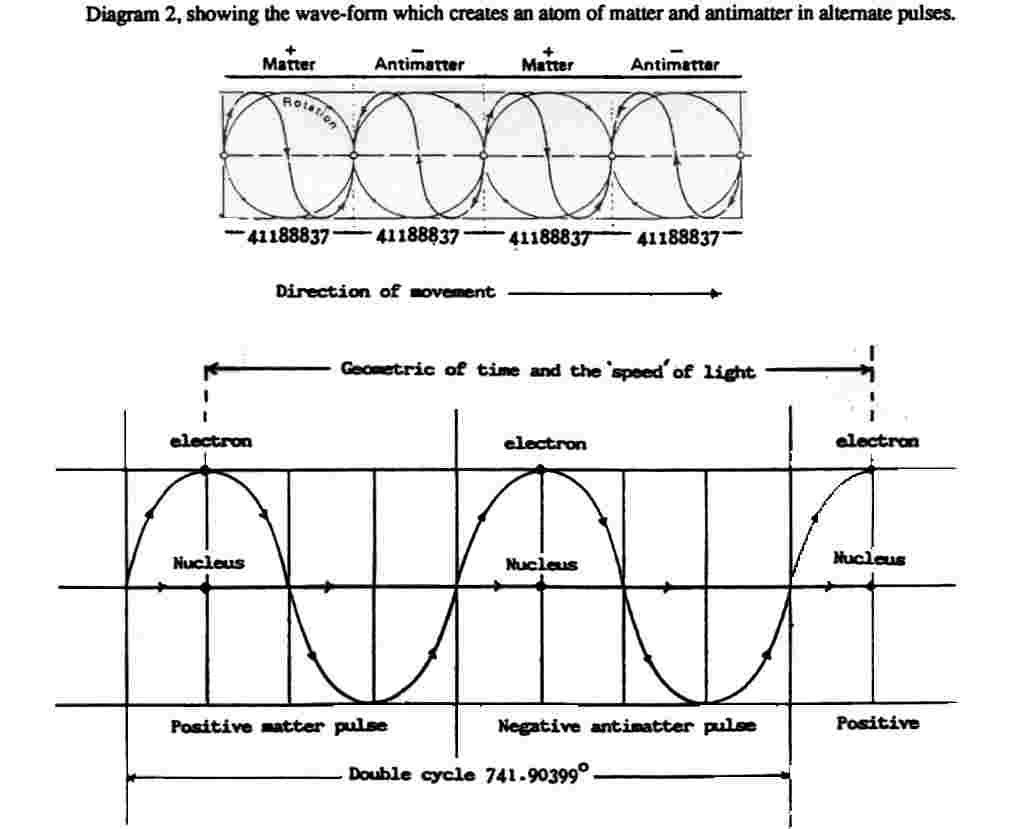Theoretically, the perception of the universal pulse, consisting of twin streams of consciousness, the matter period and anti-matter period, which are mirror reflections of each other (the positive and negative individual perception; reality and illusion), are positioned specifically in space-time. Hence, if the frequency of pulse manifestation is altered, then the perception of reality will shift in space (physical position); space travel without awareness of traversed distance; bending space. The manifestation of a light pulse occurs when the atomic structure's energy level is altered by outside influences (Max Planck).According to Cathie, the maximum number of individual elements to be found in the universe is 144, which each theoretically have 6 isotope variations (same element with different nuclear mass and atomic weight), deriving a total of 1008 (144x7=1008) separate substances. The mathematical progression between elemental octaves (original element + 6 isotopes + next higher element) derive 1152 (144x8) separate substances. The difference between the total number of substances (1008) and the harmonic value in octaves (1152) is 144, the light harmonic.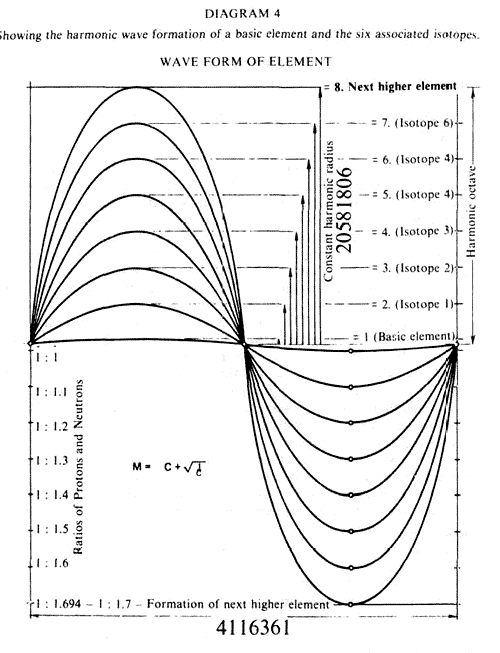The Philadelphia Experiment is the common name given to the Top-Secret US Navy experiment (1943), in which the Destroyer Escort U.S.S. Eldridge, outfitted with several tons of specialized electronic equipment capable of creating a large pulsating magnetic field itself, was made invisible and then transported from the Philadelphia Navy Yard to Norfolk Docks (400+ miles). Dr. Morris Jessup (mathematician indirectly associated with the experiment theory) said that the invisibility effect had been accomplished with magnetic field generators, called degaussers, which were pulsed at resonant frequencies to create a large magnetic field around the Destroyer. Magnetic resonance is tantamount to temporary obliteration of the perceived dimension. According to Jessup, an electric field created in a coil induces a magnetic field at right angles (spatial planes: 3 dimensions = 3 fields = 3 planes; perhaps a gravitational field); hence through the principle of resonance, it may be possible for a magnetic pulse to produce a third field (and beyond: 4th dimension; abstract mathematics theorizes 15).

According to common science, basic atomic structure is electric rather than material; the universe is a vast interplay of energy. Magnetism is thought to be the key factor controlling dimensional change/shift. Magnetic fields defy mechanical interpretation. Space travel through the modification of the space-time geometric matrix, by changing the frequencies of the matter-anti-matter wave period. According to Einstein, if the geometric of time could be altered (to zero time), then the whole universe could be accessible in a single moment. Also, that physical matter was actually a concentrated energy field, and they appeared solid because the observer consists of similar wave-forms which vibrate within a defined band-width consistent with the observer's limited perspective.

INTERNATIONAL HARMONY VIA MUSIC OF PLANETARY GRID SYSTEMS: (barbara hero)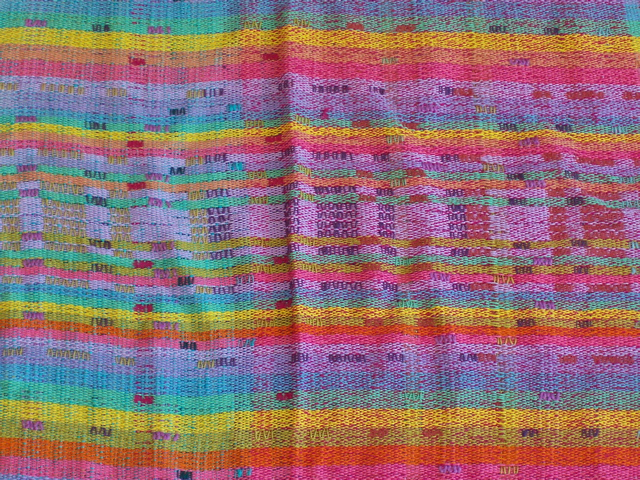Lambdoma weaving.

EARTH GRID MODULE HARMONIC THEORY:

According to Barbara Hero (collaborated with Becker-Hagens), Earth Grid systems can be correlated with specific musical notes, through a process of translating length (octave expansion and octave reduction) to frequency to musical note. After developing an Earth Grid module, which has a determined length, a musical interval could be identified. Thus, the derivation of a key note for a modular Earth grid distance between two countries in discord could potentially increase harmony. Hero's national key note correlation can be determined by means of the Pythagorean Lambdoma diagram.

Similar to Becker-Hagens, Hero realized that the Unified Vector Geometry 120 Polyhedron (including all elemental polyhedra), or the UVG 120 Earth Star, as a growing/evolving conscious geomagnetic structure which ancient people used for integrative purposes.

Considered factors for distance harmonic calculation:

• Great Circle distance (mi.) between two points on the grid system
• Major Grid Points
• Historical places as grid points
• Fault lines as energy meridians
• Mountain ranges as energy meridians
• Differences between mountains fault lines and mountain ranges
• Harmonic problems with certain distances

Harmonics of Vile Vortices:

Distances between Vile Vortices (sim.Devils' Graveyards): 4264 mi. between adjacent "Graveyards" (Robert Foulkrod finds distance with Atlas software)

Corresponding musical frequency (w/velocity through air): .0000502 cps

Through octave expansion to the middle "C" octave (x2 to the "n: number of times); trans."A"

[distance/musical note "A" may be a disharmony between sites]

Becker-Hagens EarthStar ModuleS: 30 diamond modules; 120 triangle modules

EarthStar (aka.UVG 120 Spherical Polyhedron; rhomba-triacontrahedron) = 30 Diamond Modules (30 diamond faces)

Diamond Module = 4 Triangle Modules (1 diamond face; 1/30 EarthStar; 4 Basic UVG Triangles; 4 Lambdoma matrices)

Triangle Module (aka.Basic UVG Triangle; 30-60-90 right and scalene/Pythagorean triangle) = 1/4 Diamond Module (1/4 diamond face; 1/120 EarthStar)

Pentagonal (Polar) Star = 5 diamond faces [eg. 5 diamond apexes (diamond apex = approx. 72°) mark center of pentagonal star at North Pole, radiating out to true equatorial band (ie. vile vortices)]; 5 triangles (2 Triangle Modules) arrayed from pentagonal sides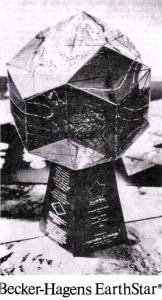Dimensions (approx.) of Triangle Module (1/4 diamond module):

Short side of triangle (line AC; 1/2 diamond width): 1400 mi. à E flat

Base side of triangle (line BC; 1/2 diamond height): 2200 mi. à A flat

Hypotenuse side of triangle (line AB; diamond side, outside length): 2600 mi. à F (345 cps); [eg.distance from Gomel (sim.Kiev), USSR to Sebha (sim.Timbuktu), Libya to generate 345 cps (F) for national harmony]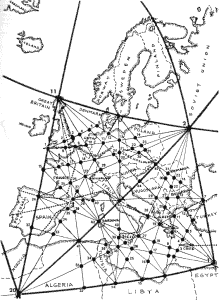[According to Sinkiewitz, the present energy grid system does not align with the ancient energy grid (eg.during Atlantean/Lemurian civilizations), and New Age spiritual communities around the world are rediscovering the new (old) grid and building/developing sacred sites for grid point activation.]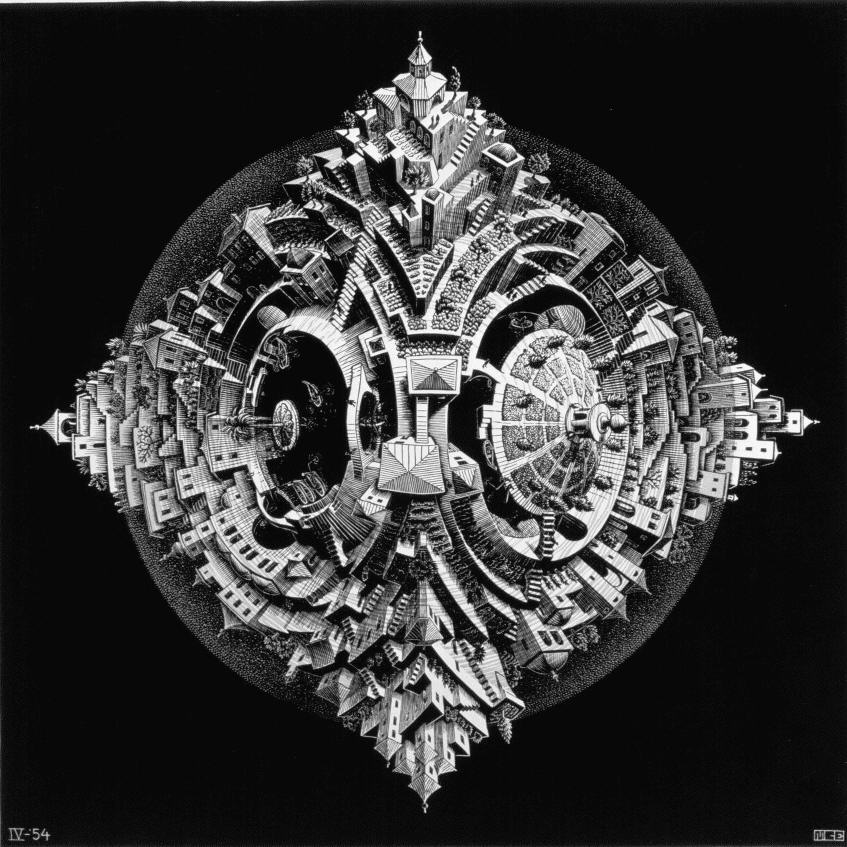Lambdoma Rhombic-Dodecahedron of M.C.Escher.

LAMBDOMA RHOMBIC-DODECAHEDRON: HARMONIC EARTH GRID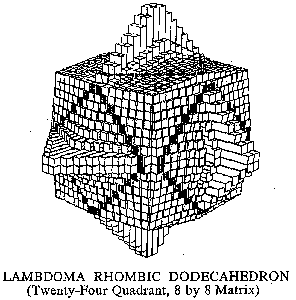Rhombic-Dodecahedron, 24(8x8) quadrant matrices, harmonically tuned to the inherent ratios of the Lambdoma Matrix.

The Lambdoma Rhombic-Dodecahedron (a diamond cube; dual-cuboctahedron) is a 12-diamond faced dodecahedron.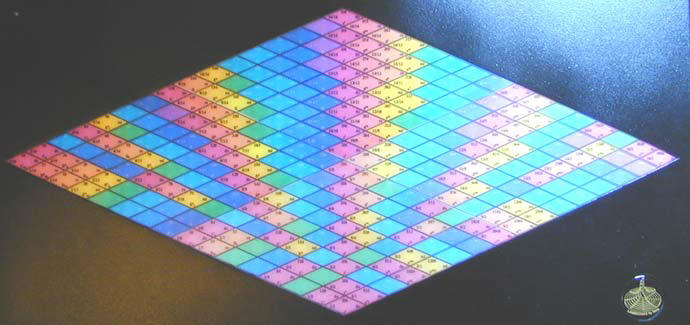A Lambdoma Harmonic expresses the sub-grid within a rhombic face of the Lambdoma Rhombic-Dodecahedron.

It is the polyhedral dual of the cuboctahedron, and a zonohedron. The long diagonal of each face is exactly √2 times the length of the short diagonal, so that the acute angles on each face measure cos−1(1/3), or approximately 70.53°; while the obtuse angle is 109.47°. (Wikipedia on 'Rhombic Dodecahedron')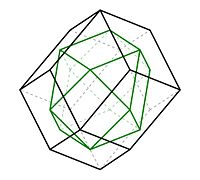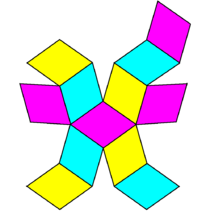The frontal view of the rhombic dodecahedron (black edges) and its dual, a cuboctahedron (green edges).

Being the dual of an Archimedean polyhedron, the rhombic dodecahedron is face-transitive, meaning the symmetry group of the solid acts transitively on the set of faces. In elementary terms, this means that for any two faces A and B there is a rotation or reflection of the solid that leaves it occupying the same region of space while moving face A to face B.  (Wikipedia on 'Rhombic Dodecahedron')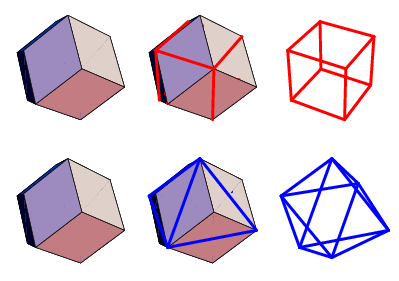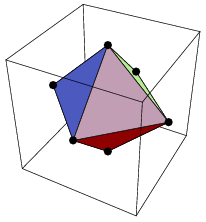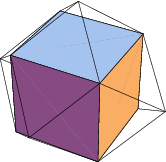The rhombic dodecahedron is one of the nine edge-transitive convex polyhedra, the others being the five Platonic solids, the cuboctahedron, the icosidodecahedron and the rhombic triacontahedron. (Wikipedia on 'Rhombic Dodecahedron')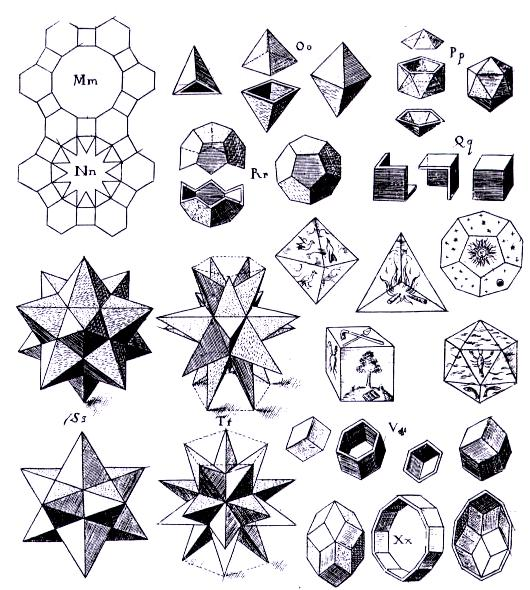Kepler's polyhedra.

From the vertex point, 3 faces are visible, while from a diamond face center of area, 5 faces are visible. The frontal view shows the true shape of one of its diamond faces; the right side view shows 6 diamond faces of 3 various perspective angles; the oblique view shows 4 squares; while the top view looks like a cube within a hexagon (also the outside perimeter of the cuboctahedron).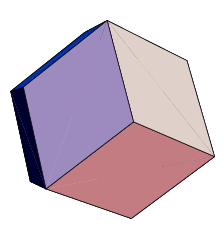The rhombic-dodecahedron, or dual-cuboctahedron, expands out of the cuboctahedron [sim. RBF vector equilibrium (VE)], through the intersection of vertex points with rhombic face center of areas, and vice-versa.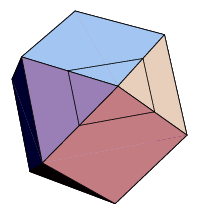Expanding the cuboctahedron [VE] into the rhombic-dodecahedron.

Honeycomb Tessellate:

Part of a Rhombic dodecahedral honeycomb

The rhombic dodecahedron can be used to tessellate 3-dimensional space. It can be stacked to fill a space much like hexagons fill a plane. (Wikipedia on 'Tessellate')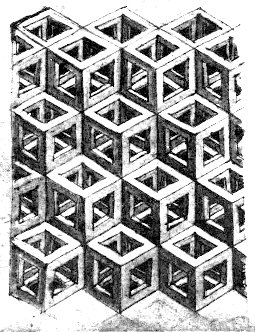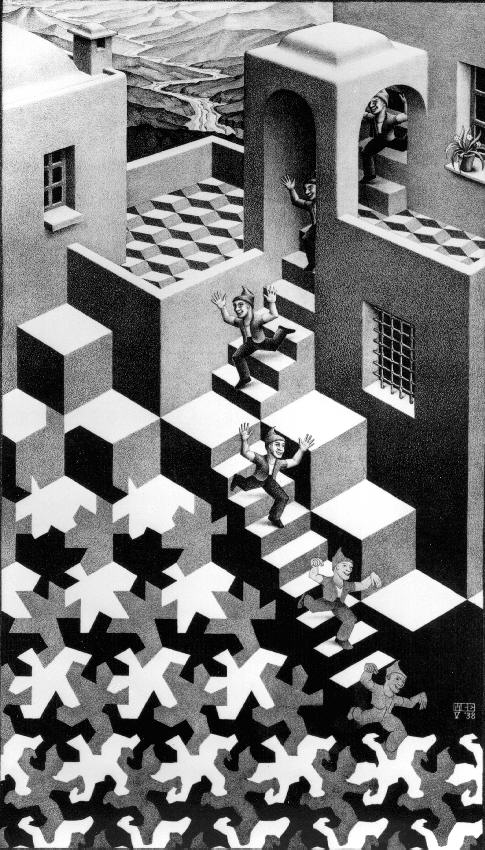Leonardo's cubes and M.C.Escher.

This tessellation can be seen as the Voronoi tessellation of the face-centred cubic lattice. Some minerals such as garnet form a rhombic dodecahedral crystal habit. Honeybees use the geometry of rhombic dodecahedra to form honeycomb from a tessellation of cells each of which is a hexagonal prism capped with half a rhombic dodecahedron.  (Wikipedia on 'Tessellate')

## Area and volume:

The area A and the volume V of the rhombic dodecahedron of edge length a are: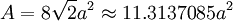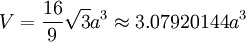## Cartesian coordinates:

The eight vertices where three faces meet at their obtuse angles have Cartesian coordinates

(±1, ±1, ±1)

The six vertices where four faces meet at their acute angles are given by the permutations of

(0, 0, ±2)

## Related polyhedra:

This polyhedron is related to an infinite series of tilings with the face configurations V3.2n.3.2n, the first in the Euclidean plane, and the rest in the hyperbolic plane.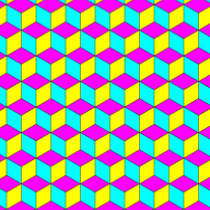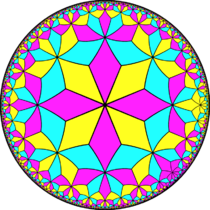Rhombic-Dodecahedron tilings.

## Related polytopes: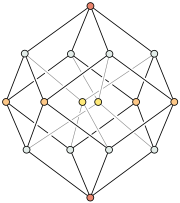In a perfect vertex-first projection two of the tesseracts vertices (marked in gold) are projected exactly in the center of the rhombic dodecahedron

The rhombic dodecahedron forms the hull of the vertex-first projection of a tesseract to 3 dimensions. There are exactly two ways of decomposing a rhombic dodecahedron into 4 congruent parallelepipeds, giving 8 possible parallelepipeds. The 8 cells of the tesseract under this projection map precisely to these 8 parallelepipeds.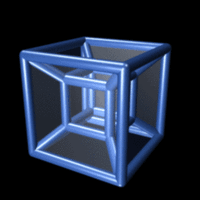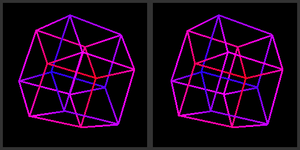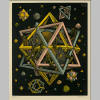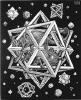Shadow of a tesseract rotating around a plane in 4D; M.C.Escher.

The rhombic dodecahedron forms the maximal cross-section of a 24-cell, and also forms the hull of its vertex-first parallel projection into 3 dimensions. The rhombic dodecahedron can be decomposed into 6 congruent (but non-regular) square dipyramids meeting at a single vertex in the center; these form the images of 6 pairs of the 24-cell's octahedral cells. The remaining 12 octahedral cells project onto the faces of the rhombic dodecahedron. The non-regularity of these images are due to projective distortion; the facets of the 24-cell are regular octahedra in 4-space.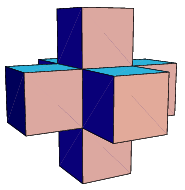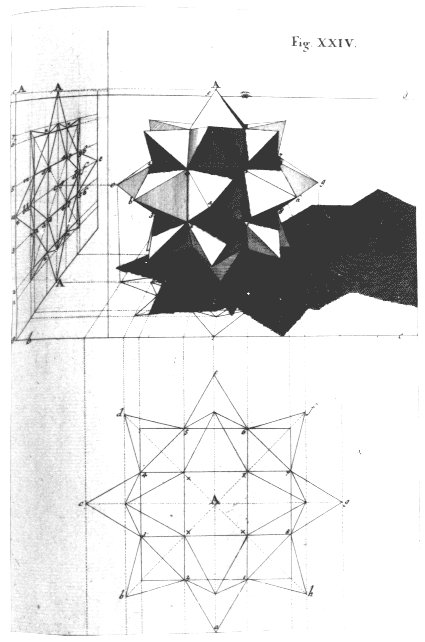This decomposition gives an interesting method for constructing the rhombic dodecahedron: cut a cube into 6 congruent square pyramids, and attach them to the faces of a second cube. The triangular faces of each pair of adjacent pyramids lie on the same plane, and so merge into rhombuses. The 24-cell may also be constructed in an analogous way using two tesseracts.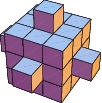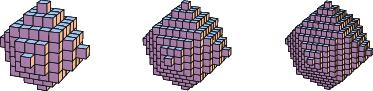(Wikipedia on 'Tesseract')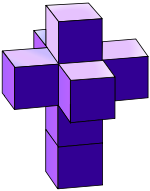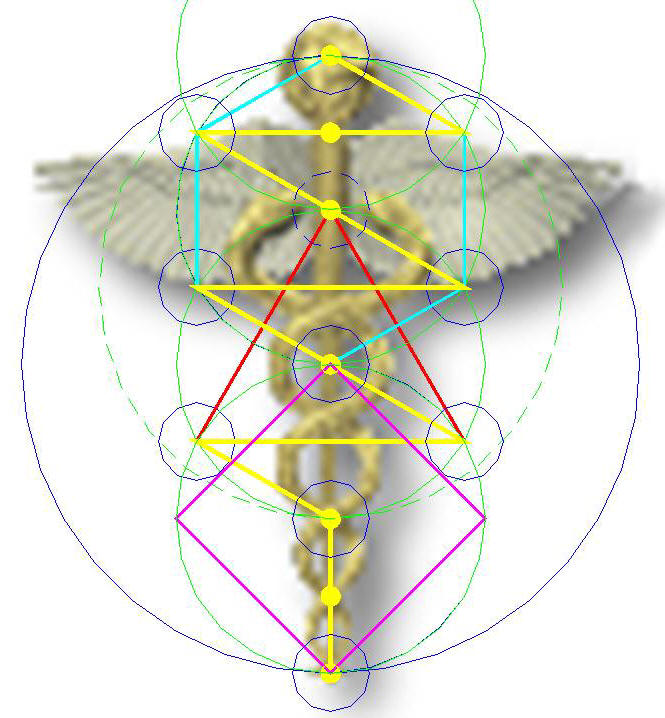The true crucifix and generic cathedral floor plan with crossing square; kaballah tree of life.

Lambdoma Matrix Face Module:The Lambdoma Matrix (see below) is a face-module (1/6) of the cube, which expands into the rhombic-dodecahedron (a diamond cube; dual-cuboctahedron). When the Lambdoma Matrix is applied to the faces of the rhombic-dodecahedron (1 matrix occupies quadrants in 4 rhombic-dodecahedron faces), its orthogonal grid becomes rhombic and skewed. The Lambdoma Matrix is the foundational Earth grid (wall paper) which pulsates sound (covers) into the polyhedral geo-energetic structure (meridian system) of the rhombic-dodecahedron and simplified into the the cuboctahedron (aka.RBF-VE) (see also cuboctahedron meridian model).3D step pyramid on face of dual-cuboctahedron [sim. Sumerian 7 staged ziggurat]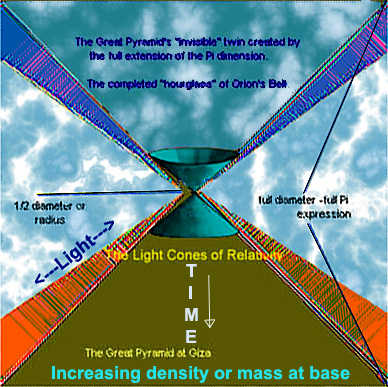pythagorean Lambdoma diagram: Foundational HaRMONIC grid COMPONENT of the RHOMBIC DODECAHEDRON FOR earth (Barbara Hero)The Lambdoma Matrix is a 16x16 harmonic grid (eg.4 chess boards/4x64 hexagrams of Yijing/4 DNA 64 codon sets of the genetic code) (see boneoracle). The Lambdoma Matrix, the foundational harmonic component of the Planetary Grid System, originates at one of the poles (N/S). [The pole is also the center point of EarthStar, a pentagon with an array of five diamond petals].

Basic Lambdoma Matrix: Greek Lamboid (4x4 Grid)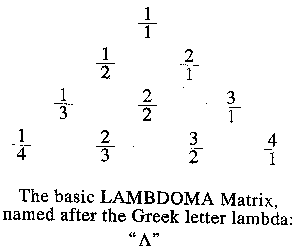In ancient Greece, the basic Lambdoma Matrix (4x4) was known as a "lambdoid" [discovered in a footnote in Nichomachus' "Introduction to Arithmetic" (trans. from Greek to Latin by the Syrian philosopher Iamblichus around 200 A.D.)]. The ratios of the right leg of the diagram represent the overtone series in music from the first (1/1) to the fourth (4/1) harmonic, while on the left leg the undertone series reveals the (1/1) to the (1/4) sub-harmonic. The former ascends in music, the latter descends (eg.Doppler Effect).When the Greek lambdoid is rotated 45 degrees into an orthogonal 4x4 matrix configuration, with x (horizontal) and y (vertical) axis, it becomes a multiplication/division table. The diagonal is then revealed to be always a 1:1 ratio, which represents a fundamental frequency of the same musical note, or a "constant."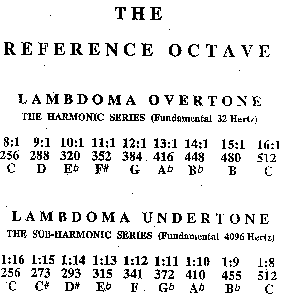The reference octave defines middle range octave frequencies of the overtone (fundamental: 32 cps) and undertone (f: 4096 cps) scales, expressing the differences between ascending and descending scales of the Lambdoma Matrix. These scales begin at the 8th harmonic overtone and the 8th sub-harmonic undertone, forming an 8 note scale rather than a 7 note scale within a 16x16 matrix.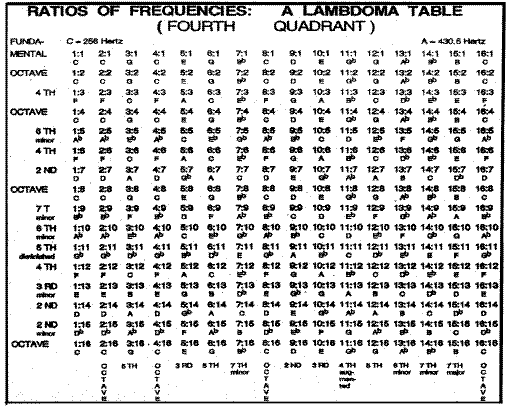The Lambdoma Matrix (16x16 grid; above), encompasses a full 256 variation entry musical microtonal array, or 256 ratios of frequency [measured: cycles per second or Hertz (Hz)]. A microtonal variation becomes distinctively audible (a musical note) after expressing a 1:1 ratio of frequency, fundamentally found between the 256-512 Hz range. A 1:1 relationship of the overtone/undertone music series becomes apparent from the ratio to musical interval notation within a (16x16) Lambdoma matrix.

Table shows numerical relationships (ratio) of whole numbers to specific music intervals. Reading across rows from left to right results in an undertone series, while reading up the columns, beginning at the lower left, results in an overtone series. Superscripts indicate the octave above the middle C, while subscripts indicate the octave below the middle C.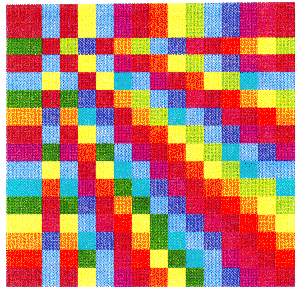Both Pythagoras and Isaac Newton color-coded musical scales in the following way:

[ie.C (red), D (orange), E (yellow), F (green), G (blue), A (indigo) and B (purple)].

However, when one raises the frequencies in music to the 14th power the note-colors become complementary:

[ie. C (green), D (blue-green), E (blue), F (purple), G (red), A (orange), and B (yellow)].

Musical sounds (measured in frequencies), and colors (in wavelengths) have an inverse mathematical relationship.

It seems logical to measure both in terms of frequency, which then unties the Mobius frequency/wavelength strip from a half turn to an untwisted flat strip.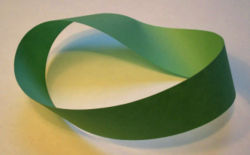The Möbius strip or Möbius band (pronounced or /ˈmoʊbiəs/ in English, IPA: [ˈmøːbiʊs] in German) (alternatively written Mobius or Moebius in English) is a surface with only one side and only one boundary component. It has the mathematical property of being non-orientable. It is also a ruled surface. It was discovered independently by the German mathematicians August Ferdinand Möbius and Johann Benedict Listing in 1858.

A model can easily be created by taking a paper strip and giving it a half-twist, and then joining the ends of the strip together to form a single strip. In Euclidean space there are in fact two types of Möbius strips depending on the direction of the half-twist: clockwise and counterclockwise. The Möbius strip is therefore chiral, which is to say that it has "handedness" (as in right-handed or left-handed).

It is straightforward to find algebraic equations the solutions of which have the topology of a Möbius strip, but in general these equations do not describe the same geometric shape that one gets from the twisted paper model described above. In particular, the twisted paper model is a developable surface (it has zero Gaussian curvature). A system of differential-algebraic equations that describes models of this type was published in 2007 together with its numerical solution.

The Euler characteristic of the Möbius strip is zero.

(Wikipedia on 'Mobius')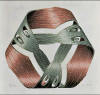M.C.Escher

Shape-Coding: Wave Form for Variation in Ratio of Frequency

Lissajous Figures: Trigonometric Arc-Tangent Designation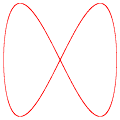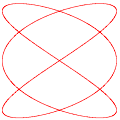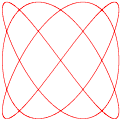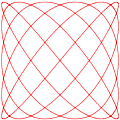In mathematics, a Lissajous curve (Lissajous figure or Bowditch curve) is the graph of the system of parametric equations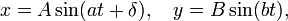which describes complex harmonic motion. This family of curves was investigated by Nathaniel Bowditch in 1815, and later in more detail by Jules Antoine Lissajous (pronounced LEE-suh-zhoo) in 1857.

(Wikipedia on 'Lissajous')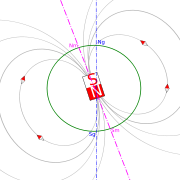Lissajous figures were computer generated by a trigonometric arc-tangent program, which also included a color-coded 9x12 matrix based upon the fundamental frequency of 480 cps of the color purple, which we would hear as a B in music.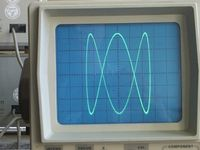Prior to modern computer graphics, Lissajous curves were typically generated using an oscilloscope.

Shape-coding by Lissajous figures expresses a trigonometric arc-tangent designation for a variation in ratio of frequency within the Lambdoma Matrix. As in the previous color-coding, all the octaves (doublings) of each of the eight note musical scale are depicted in the same color.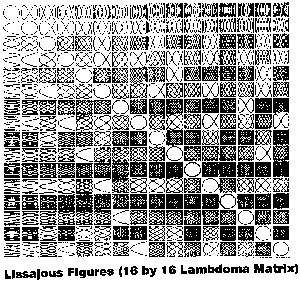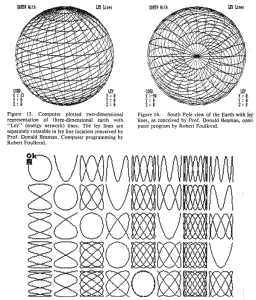A shape coding of Lissajous figures in black and white of a 16x16 Lambdoma Matrix indicates the concept of interval (two notes sounding together). Note how the fundamental frequency 1:1 through n:n of any two notes of the same pitch becomes the circular shape, which runs down the diagonal.

Sound Images:

• Sounding two notes of exactly the same pitch in frequency (cps; eg.256:256 Hz) together: sound would appear as a circle
• Sounding two sounds exactly an octave apart (eg.doubling 256:512): sound would appear as a figure eight.

The (5x8) Lambdoma Matrix with ratios expressed by Lissajous figures (patterns formed by whole number ratios between a fundamental tone, overtone, and undertone) represents an Earth grid evolution as defined by Pythagorean intervals in sound.A computer generated "Lambdoma Harmonic Keyboard" is a prototype for a musical instrument, which plays the music of the Lambdoma Matrix. A laser scanner auxiliary system displays the Lissajous figures on a screen or wall as the sounds are played. Overlays indicating color, octaves, ratios, musical note and frequency, based on a fundamental of 256 cps, is attached to the diamond shaped keyboard; (shape-coding overlays can also be applied).

Colored Symbols: Ancient Pictographic Languages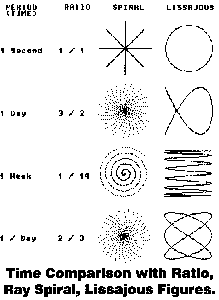In this Shape-Coding, the fundamental frequency would be a C at 256 cps because of the red star-like shapes of the diagonal.

A colored symbol designation for variation in ratio of frequency [sim.ancient pictographs: native American, Egyptian, Sumerian] within the Lambdoma Matrix, may suggest the harmonic intention for language.

Binary Cosmic Code: Yijing Oracle Divination and 256 Sound Frequencies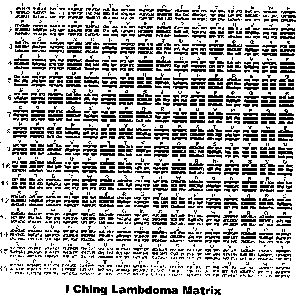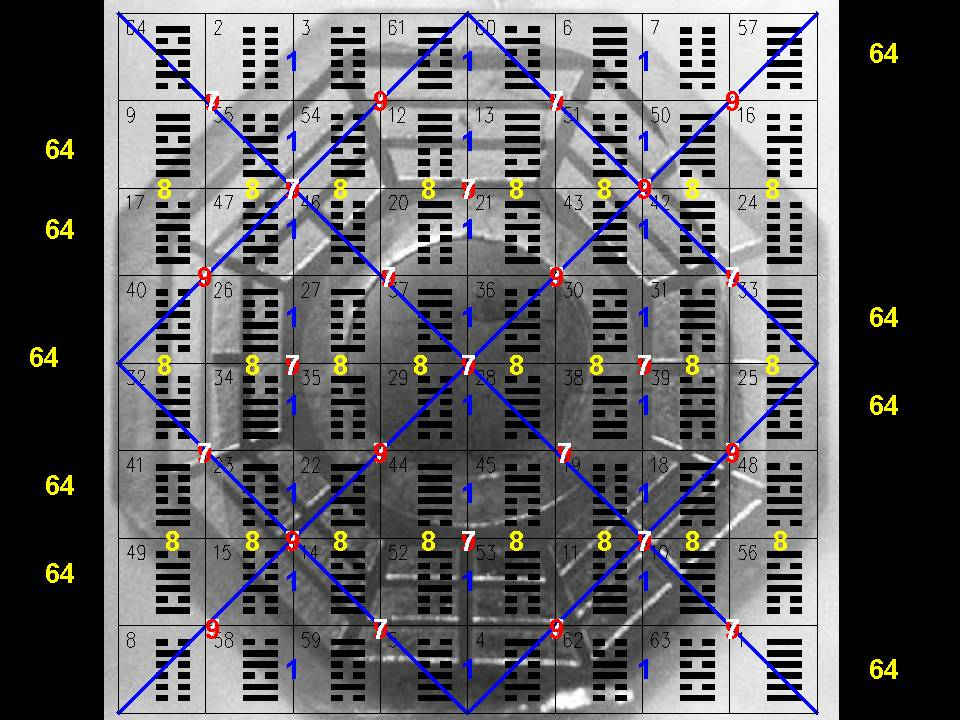Magic Square of Mercury superimposed on the 64 hexagrams; note intervals of 7 on the diagonals.

The Lambdoma Matrix contains 4(8x8) grids of Yijing Trigrams, which derive the 64 Hexagrams [sim.chess board; DNA 64 codon sets of the genetic code] (see boneoracle), hence deriving a Yijing oracle from one of 256 (16x16) musical sounds (eg.Chinese trans.: Yijing divination = divine oracle message).

Architectural Matrix: 3D Model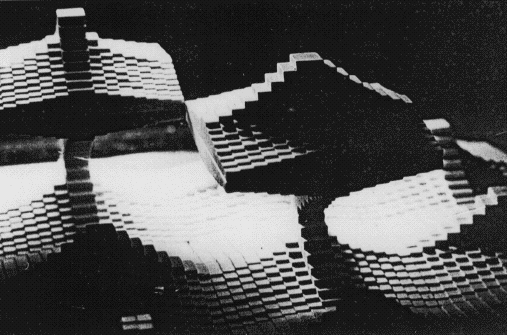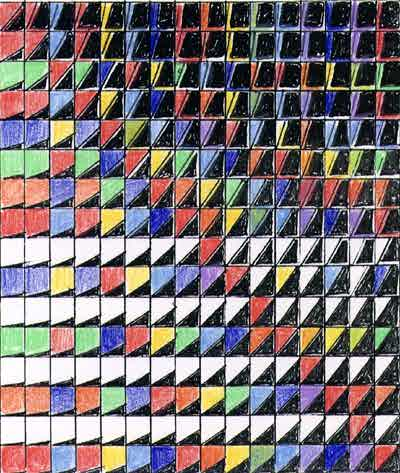Architectural type 3D Lambdoma matrices, based upon wavelengths instead of frequencies, expresses four quadrants representing lengths of different ratios, which can be translated into any dimension of distance (eg.multi-level dwellings), in harmonic accordance. Each isometric square has a fundamental unit of measurement, which represents a constant fundamental frequency in cycles per second.

Round Model: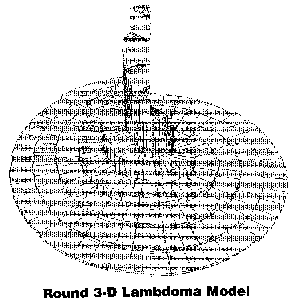The ratios of the Lambdoma Matrix transformed into a round architectural model (eg.urban design on earth, water, or air).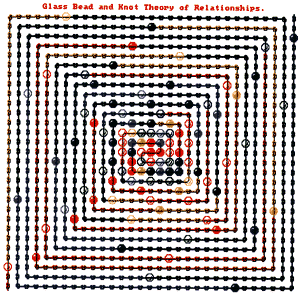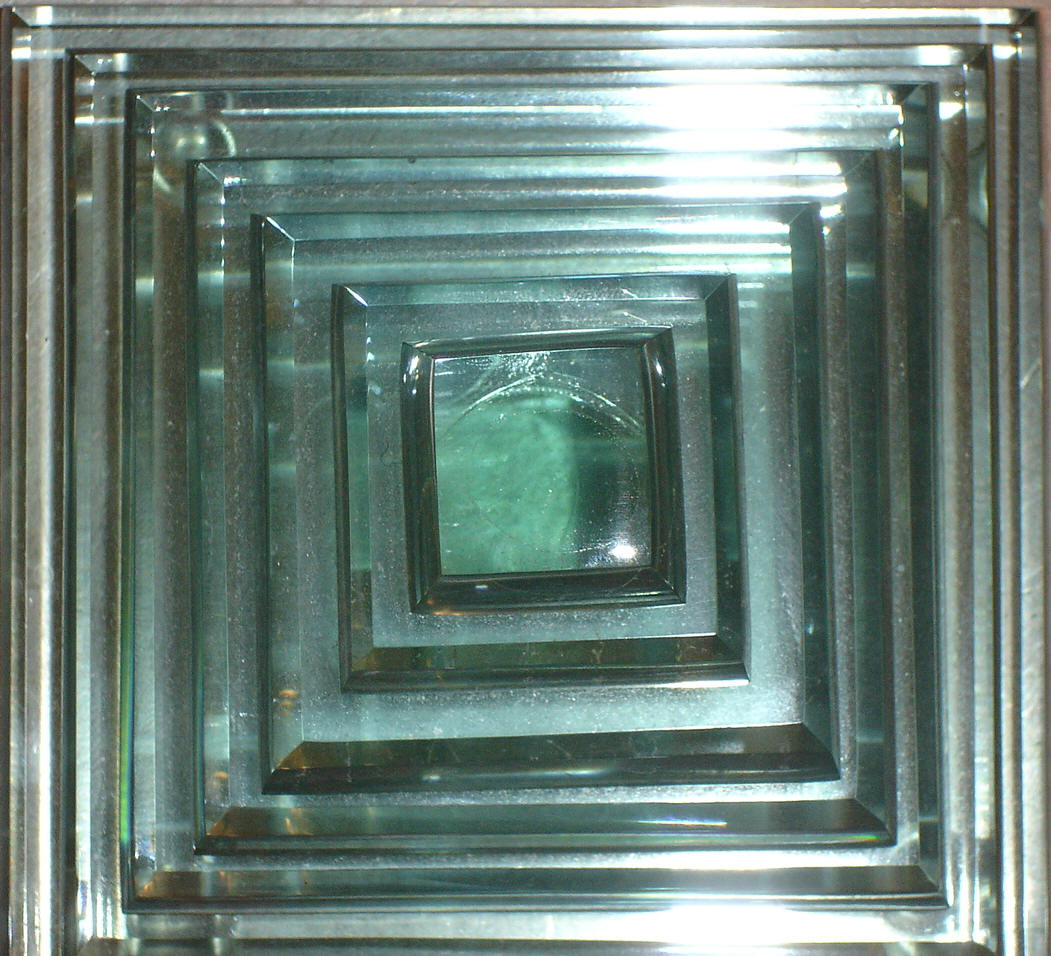This computer generated necklace model was based on the book by Hermann Hess "Magister Ludi, the Glass Bead Game", where it seemed that the Lambdoma Matrix might be a template for illustrating the game. The beads which represent the harmonic series begin at the center, where each bead represents a musical note-color up to the 16th harmonic. The series of 8 beads is then separated by small beads for five times marking the five octaves. At each octave the patterns between the 8 larger beads becomes 1, 3, 7, 15, etc. The beginning fundamental is the note C which is red. One can trace each of the octave reds as they become more and more sparsely distributed after they complete their 8 note cycle.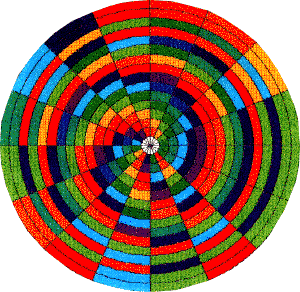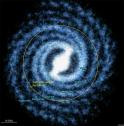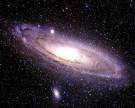The period of the orbit of the sun around its galaxy (about 25,000 years) is depicted by a Lambdoma mandala. The music of the fundamental may be heard by translating period of the orbit to the frequency of 332.8 an E+ note, in the reference octave. The color of yellow-green marks the beginning of the computer generated matrix on the right side of the outer circumference.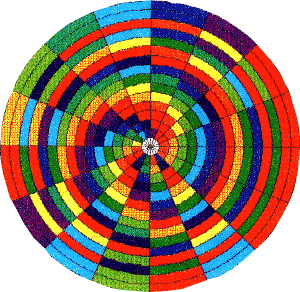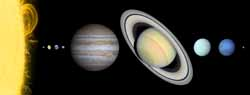The period of the orbit of the earth around the sun of 365.25 days is portrayed by translating its period of rotation into 272.6 cycles per second, a C#/Db musical note. This concept was then computer generated into a Lambdoma mandala. The overtone progression of the Lambdoma begins at the right hand circumference of the mandala with the color red.

DERIVATION OF SOUND FREQUENCY FOR DISTANCES:

Formula for determining the frequency of a distance: v = fw

(v)elocity through medium (ft/sec): velocity of sound in air at room temperature in ft/sec = (1130)

(f)requency in cycles/sec (cps) or Hertz (Hz): measures sound

(w)avelength or distance in feet (ft) [5280 ft = 1 mi.]: measures light

Due to the nature of the inverse properties of frequency (measurement of sound) and wavelength (measurement of light), frequency (f) or wavelength (w) can be determined if the velocity (v) of sound traveling through a particular material is known.

The measurement of light (in wavelength) and sound (in frequency) have separate relationships (velocity to density); but are within the same electro-magnetic spectrum (see also Bloch Wall):

Sound Frequency (f): sound velocity decreases (travels slower) as medium material density decreases [sound velocity directly relates with density]; ultimately stopping and transforming into light within a transparent least dense medium [decreasing velocity: earth-water-air-vacuum; earth to heaven (eg.sound would stop traveling and transform into light within a hypothetical Hollow Earth)]

[birdsong voice becomes light]

Light Wavelength (w): light velocity decreases (travels slower) as medium material density increases [light velocity inversely relates with density] ; ultimately stopping and transforming into sound within a non-transparent, most dense medium [decreasing velocity: vacuum-air-water-earth; heaven to earth]

[divinity speaks oracle]

Illustration for Deriving Harmonic Mean:

1) Distances: take/find a distance (mi.) (A) between point a (first/original site) and point b (second site), then take/find another distance (B) from either point a/b to another point, point c (third site)

2) Sum of Distances: put the resulting lengths end to end (total: A+B; length of line a-b, and line b-c, or a-c)

3) Circle construction: bisect the total length (A+B/2) and draw a circle with its center at the midpoint

4) Geometric Mean: draw a line orthogonal (90°) to the diameter of the circle from point a (where this line intersects the circle determines the geometric mean

5) Arithmetic Mean: draw a line from the geometric mean intersection point to the center of the circle to determine the arithmetic mean (radius of circle)

6) Harmonic Mean: draw a line orthogonal to the radius, extending to point A (original site) to determine the harmonic mean

[All derived distances can be translated into sounds (harmonic notes), which would be harmonious with all points in question.]

[eg.Multiple electro-magnetic geometric patterns, impositions from within/without the Earth (eg.planetary geometaphysics; stars, Sun, Moon, planets; creative intelligence, or the origin of will power), influence the rhythms of civilizations on the surface range.]

determination of key note: APPLICATION OF LAMBDOMA WITH FREQUENCY DIAGRAM

Each grid vector (eg.ray in question) exhibits a unique energy (frequency = key note)

[eg.Frequency of Earth: circumference of Earth: 24,860 mi. = Key Note "D"].Top: Spinning Earth with Ley lines from equatorial and polar views; Bottom: The 5x8 Lambdoma Matrix with ratios expressed by Lissajous figures (patterns formed by whole number ratios between a fundamental tone, overtone, and undertone) represents an Earth grid evolution as defined by Pythagorean intervals in sound (Prof.Donald Beaman and Robert Foulkrod).

A key note of a country is determined by means of the Lambdoma Diagram of Pythagoras. The apex of the Lambdoma (rhombic dodecahedron; a 12-diamond faced dodecahedron), is divided equally as the diagonal (z axis) down the center point of the x and y axis. The diagonal has a ratio of 1:1; so the distance (length) of the grid diagonal generates frequency (cps), its key note.

[eg.Gomel-El Eglab (near Timbuktu) = 2600 mi. = F; Cairo-London = 2183 mi. = G#]Table of Frequencies and Musical Notes Relative to Miles in Air: easy derivation of musical note from distance in miles.

Conclusion:

The (5x8) Lambdoma Matrix with ratios expressed by Lissajous figures (patterns formed by whole number ratios between a fundamental tone, overtone, and undertone) represents an Earth grid evolution as defined by Pythagorean intervals in sound. If Earth grid transforms in phases, and is in structural transition (a change in distance), then frequency change at different locations may result.

For humans to establish the anthropocosm (cosmic human, true human), they need to tune (observe/listen) into each other's energy pattern frequencies (eg.human integrity: peace), recognize (discover) harmony with the Earth (eg.look: material integrity: oneness with the universe; following nature; using free will to follow destiny), and know (create) the importance of sound as a manifestation of different realities (eg.cosmic integrity: create harmonic sound). Any grid system has a distance factor, since wavelength is inherent in all sound vibrations as the inversion of frequency. The specific frequency, or interval quality, of a grid line is inaudible, but present.